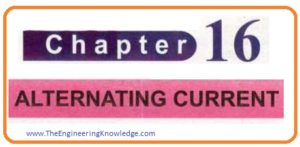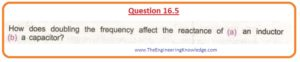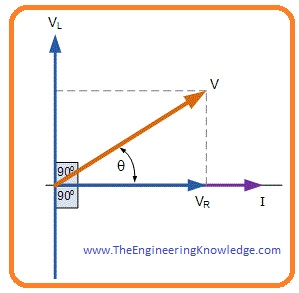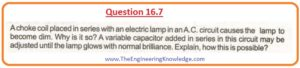Hello dear FSC students, I hope you all are doing great. In today’s tutorial, we will have a look at 2nd Year Physics Chapter 16 Solved Questions. I have started a series of tutorials related to the solution of questions given in 2nd-year physics. In the previous tutorial, I have discussed all the questions of chapter15 with the detailed.

In this post, we will have a detailed look at all the questions given in chapter 16 of FSC physics. I will explain all the questions one by one and give an answer to every question in a simple way. So let’s get started with 2nd Year Physics Chapter 16 Exercise Solved Questions.

#### 2nd Year Physics Chapter 16 Exercise Solved Questions

• Let’s friends discuss all the questions given in chapter 16 with the detailed.

#### Question 16.1• As we have given that.

Irms= 10 ampere

We have to find peak current or Io

Irms= Io/√2

Io= Irms .√2

=10 . (1.414)

Io =14.14 Amperes

#### Question 16.2• The inductor is a device that stops the flow of alternating current and allows the DC current to flow.
• The capacitor is a device that allows the AC to flow but stops the DC to flow.

#### Question 16.3• For one cycle Alternating current gets the maximum value 2 times. So for fifty cycles per second, it will get maximum brilliance a hundred times.

(2) . (50)=100 time in one second

#### Question 16.4• When we close the witch than a path for flow of current completed and due to flow of current magnetic field will produce in the circuit.
• The energy stored in the inductor will be given here.

Um=1/2(LI2)

• When we open the switch circuit will be open and energy stored in the inductor will be lost an will be shown in the form of flash across the contacts of the switch.

#### Question 16.5(a):

• As we know that reactance of the inductor is (XL= 2πfL)
• From the above equation, you can observe that XL is directly proportional to frequency.
• So if we double the frequency inductive reactance will also be doubled.

(b):

• While for capacitor formulas of capacitor reactance is XC = (1/2πfC).
• From the above equation, you can see that capacitive reactance is inverse proportional to the frequency.
• With the doubling of frequency capacitive reactance will become half.

#### Question 16.6• In the below figure you can see that voltage V leads the current by an angle ‘θ’.tan θ = (ωL/R)
or θ  = tan(-1)(ωL / R)

#### Question 16.7• When in any circuitry choke coil is connected in series with the bulb then due to inductance the XL (inductance reactance) will have a large value.
• Due to that small amount of current will flow through the circuit. The bulb will glow dim.
• When we linked the variable capacitor in series the circuit will become RLC series resonance circuitry.
• By varying the value of capacitor resonance frequency can be adjusted to equals to the frequency of input alternating current source.
• At this point, resonance occurs and the circuit’s impedance will become less.
• Due to this condition, large amount of current will flow through the circuitry and bulb will glow in normal brightness.

#### Question 16.8• When an antenna is attached with the alternating current source it accelerates electrons up and down along the wire..
• Due to that polarity of end terminals of the antenna will changes.
• If a charge is positive for first half cycle and after second half will be negative charge.
• Due to this variable electrical field will be generated in results that will produce a magnetic field.
• Due to that electromagnetic waves propagates.

#### Question 16.9• The specific radio channel will be tuned if the frequency of local oscillator or LC circuit is similar to the frequency of electromagnetic waves transmitted by the specific station.

f=1/(2Π√LC)

or  f ∝ 1/√C

• For a variation of frequency of LC circuit can be done by varying the capacitance of a variable capacitor.
• When this frequency is similar to the frequency of an incoming signal of the station to be tuned resonance will occur and extreme response will be obtained.

#### Question 16.10• The AM stands for amplitude modulation. In this transmission mode amplitude of carrier, a signal is varied but its frequency remains constant.
• Its frequency range is from 540 kilohertz to 1600 kilohertz.
• The FM stands for frequency modulation. In this transmission mode frequency of carrier wave is varied but amplitude remains constant.

#### Related Posts

So, friends, it is the detailed post about the 2nd Year Physics Chapter 16 Exercise Solved Questions if you have any queries about this post ask in comments. See you in next post have a good day. Soon I will share the solution of chapter 17 exercise question.

### 1 Comment

1. Online Scams says:

May I just say what a relief to find someone who actually understands what they’re talking about on the internet.

You actually realize how to bring a problem to
light and make it important. More people need to look at this and understand this side of your story.
I can’t believe you’re not more popular because you definitely possess the gift.